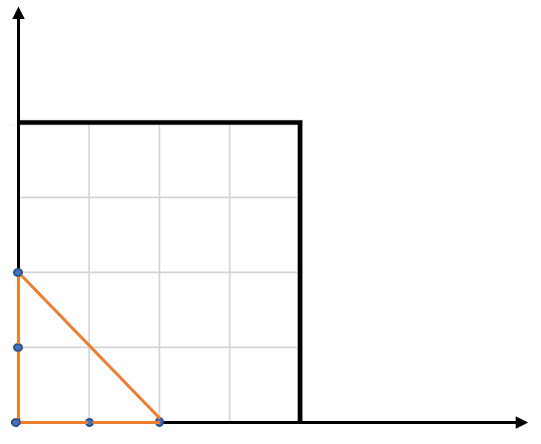## 题目描述：

LeetCode 812. Largest Triangle Area

You have a list of points in the plane. Return the area of the largest triangle that can be formed by any 3 of the points.

```Example:
Input: points = [[0,0],[0,1],[1,0],[0,2],[2,0]]
Output: 2
Explanation:
The five points are show in the figure below. The red triangle is the largest.
```Notes:

• `3 <= points.length <= 50`.
• No points will be duplicated.
•  `-50 <= points[i][j] <= 50`.
• Answers within `10^-6` of the true value will be accepted as correct.

## 解题思路：

Shoelace formula （根据顶点坐标求三角形面积）

## Python代码：

``````class Solution(object):
def largestTriangleArea(self, points):
"""
:type points: List[List[int]]
:rtype: float
"""
triangleArea = lambda x1, y1, x2, y2, x3, y3: \
abs(0.5 * (x1 * (y2 - y3) + x2 * (y3 - y1) + x3 * (y1 - y2)))
size = len(points)
ans = 0
for i in range(size):
for j in range(i + 1, size):
for k in range(j + 1, size):
x1, y1 = points[i]
x2, y2 = points[j]
x3, y3 = points[k]
ans = max(ans, triangleArea(x1, y1, x2, y2, x3, y3))
return ans
``````

Pingbacks已关闭。